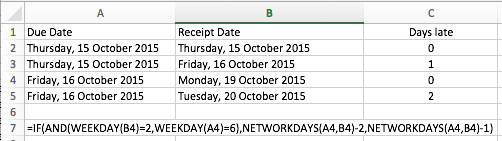# How to identify weekends in excel tables

2 posts / 0 new
How to identify weekends in excel tables

I wish to identify weekends in excel table to identify late shipments from suppliers. i.e. where a supplier delivers on due date [say a Friday] but inwards goods receipts on the following Monday, I want a quick and easy way to identify the intervening weekend rather than excluding all 2 day "late" receipts.
Is that possible?

Hi GSJ

One approach you could take would be to use the WEEKDAY() function to calculate what day it is, and the NETWORKDAYS function to calculate the number of working days between two dates (assuming Saturday and Sunday are weekend days).

The WEEKDAY funciton returns a value between 1 and 7, where Monday would be 2. So you could write a formula that checks whether the receipt day is Monday AND the date due was Friday. In this case you would calculate the Days Late as zero. In any other circumstance, the Days Late would be the difference between the due date and the delivery/receipt date.

Here's an example based on that approach:This example shows four scenarios, two of which would be calculated as "Late".

The two Friday scenarios show the calculated late days for Friday deliveries. The formula below shows how this is done:

• We use an IF function to determine whether the Receipt date was a Monday (weekday = 2) and the due date was a Friday (weekday = 6).
• If this is true, then we calculate the NETWORKDAYS between the Due Date and the Receipt date, then subtract 2 from the result.
• The NETWORKDAYS function calculates the different between two dates, and subtracts weekend dates before returning the result.
• However, it includes the start and end dates in the calculation, so we need to subtract 2 days to correct for this.
• If this is not true (i.e. either the Due Date wasn't a Friday OR the Receipt date wasn't a Monday) then we use the NETWORKDAYS function again, but only adjust by one day.

I hope that helps, or at least gives you a few new ideas to work with in solving your problem.